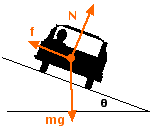# Car on the banked turn with friction

## Homework Statement

Car traveling along the "reverse" banked turn (road tilted away from the curvature). Radius of curvature is 15 m, static friction between road and tires is 0.5, and banking angle is 10 degrees. Find the maximum velocity possible on this reverse banked turn.v=sqrt(Usgr)

## The Attempt at a Solution

sqrt(0.5 x 9.8 x 15)= 8.57 m/s

Its wrong as actual answer is 7.2m/s

#### Attachments

Last edited:

i get 7.8m/s..

i get 7.8m/s..

how?

I have tried and got answer using this equation:
v=sqrt(((MUcostheta-sintheta) x g x r)/(costheta-MUsintheta))

I derived it by:
sum of x forces: Fx=Fcostheta-MU(Nsintheta)=(mv)^2/r
sum of y forces: Fy=0=Ncostheta-MU(Nsintheta)-mg
mg=N(costheta-MU(sintheta)
N=(mg)/(costheta-MU(sintheta))

Netforce: -Fnet(opposite direction)=Nsintheta-fcostheta(Opposite direction)
Fnet=-Nsintheta+MU(Ncostheta)
=N(-sintheta+MU(costheta))
=((mg)/(costheta-MU(sintheta)) x (-sintheta+MU(costheta)
Fnet=Fcentripetal

(mv^2)/r=((mg)/(costheta-MU(sintheta)) x (-sintheta+MU(costheta)
v=sqrt((MUcostheta-sintheta) x g x r)/(costheta-MUsintheta))

Last edited: